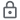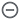# Fractions and Odds Calculator

10K+Everyone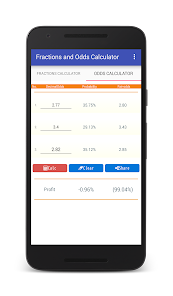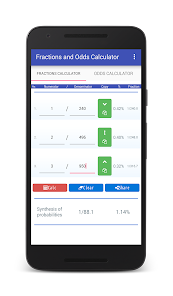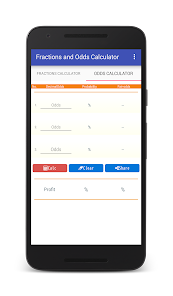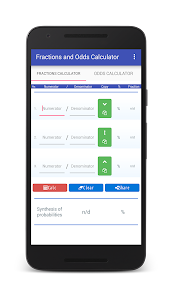You can calculate multiple fractions.(Up to three)
You can also calculate with the same denominator and different denominators with a single button.And you can calculate three odds and calculate fair odds.It can be used for phase odds calculations such as sports books.

"Fractions Calculator"tab
Composite calculation of up to three fractions.
Enter the numerator and denominator in each row, and press the "Calculate" button.
In the column of "Synthesis of probabilities", we display the fraction and the probability of synthesis, expressed as a percentage.

"Odds Calculator"tab
Calculate up to three odds.
Please enter the odds on each line and press the "Calculate" button.
- In the "Probability" column, the probability of being considered to occur with that odds is displayed.
- In the "fair-odds" column you will see the correct odds calculated from that odds combination and probability.
- In the "Profit" column, a positive value will be displayed if it exceeds 100%.
It can be used for calculating fair odds such as sports books.

Use the "Clear" button to erase all inputs.
By "Share" button you can record it in Notepad etc.

== Recommended operating environment ==
Android 4.0.3+

This application does not handle user information.
http://androidkaihatu.blog.fc2.com/blog-entry-97.html
Updated on
Dec 18, 2021

## Data safety

Safety starts with understanding how developers collect and share your data. Data privacy and security practices may vary based on your use, region, and age. The developer provided this information and may update it over time.No data shared with third parties